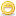CodeGuru Home VC++ / MFC / C++ .NET / C# Visual Basic VB Forums Developer.com

1.Junior MemberJoin Date
Feb 2011
Posts
3

##Rand() function

I'm trying to make a simple console game using Microsoft visual c++ 2010. It is a guessing game in which it creates a random number and you try to guess the number. There are two options, 1 to 10, or 1 to 100. The problem is it keeps generating a new random number every time i guess. I need the number to stay the same throughout the game. Does anyone know what the problem is?

Here is my code:

Code:
```#include <iostream>
#include <time.h>

using namespace std;

void game1();
void game2();

void main() {
cout << "Guess the number" << endl;
cout << "----------------" << endl;
cout << "Please select an option" << endl;
cout << "1. 1 to 10" << endl;
cout << "2. 1 to 100" << endl;
int selection;
cin >> selection;
if (selection == 1) {
game1();
}
if (selection == 2) {
game2();
}
}

void game1() {
system("cls");
cout << "Guess the number (1 to 10):" << endl;
for (int times = 1;;times++) {
int guess;
int number;
srand(time(0));
number = int(rand() &#37; 10 + 1);
cin >> guess;
if (guess == number)
{
system("cls");
cout << "You win!" << endl;
cout << "It took you " << times << " times to guess." << endl;
cout << "Would you like to play again? (y/n)" << endl;
game1();
}
main();
}
system("pause");
}
else
if (guess > number) {
cout << "The number is less than " << guess << ". Guess the number (1 to 10):" << endl;
}
else
if (guess < number) {
cout << "The number is more than " << guess << ". Guess the number (1 to 10):" << endl;
}

}
}

void game2() {
system("cls");
cout << "Guess the number (1 to 100):" << endl;
for (int times = 1;;times++) {
int guess;
int number;
srand(time(0));
number = int(rand() % 100 + 1);
cin >> guess;
if (guess == number)
{
system("cls");
cout << "You win!" << endl;
cout << "It took you " << times << " times to guess." << endl;
cout << "Would you like to play again? (y/n)" << endl;
game2();
}
main();
}
system("pause");
}
else
if (guess > number) {
cout << "The number is less than " << guess << ". Guess the number (1 to 100):" << endl;
}
else
if (guess < number) {
cout << "The number is more than " << guess << ". Guess the number (1 to 100):" << endl;
}

}
}```
Last edited by Marc G; February 22nd, 2011 at 04:15 AM. Reason: Added code tagsReply With Quote

2.Member +Join Date
Jul 2009
Location
India
Posts
835

## Re: Rand() function

1st of all use CODE tags around your code and 2nd thing is its a VC++ forum. Post all your further queries relates to C++ in this forum.

And the solution is to use a 'static variable'. Though I didn't go through your code just posted the ans by reading the txt.Reply With Quote

3.MemberJoin Date
May 2006
Location
Dresden, Germany
Posts
458

## Re: Rand() function

Hi,

Please use code tags when posting code (see FAQ's for details)!

srand is menat to be called once (at the start of your program). It initializes the pseudo random generator.

rand itself can be called as often as you want. In case it should be called exactly when you need a new random number. Then you can hold this value in a variable and make your guesses until you'll have got it.

With regards
ProgramartistReply With Quote

4.Junior MemberJoin Date
Feb 2011
Posts
3

## Re: Rand() functionReply With Quote

5.Elite Member Power PosterJoin Date
Nov 2003
Location
Florida
Posts
12,624

## Re: Rand() function

Also, never, and I mean NEVER call main directly. Never.

You need to start using loops instead of recursive calls as you're doing. That's really bad design.

Also, if there's no reason for two different game functions. All you need is one function that knows the upper limit.Reply With Quote

6.Elite Member Power PosterJoin Date
Nov 2003
Location
Florida
Posts
12,624

## Re: Rand() functionOriginally Posted by hypheni1st of all use CODE tags around your code and 2nd thing is its a VC++ forum. Post all your further queries relates to C++ in this forum.

And the solution is to use a 'static variable'. Though I didn't go through your code just posted the ans by reading the txt.
There's no need for a static variable here. A local variable in the game function would suffice. He just needs to call rand outside of the guessing loop.Reply With Quote

7.Junior MemberJoin Date
Feb 2011
Posts
3

##Re: Rand() function

Thank you for your help GCDEF. I did what you said and it worked.Reply With Quote

console, game, number, rand, random####Posting Permissions

• You may not post new threads
• You may not post replies
• You may not post attachments
• You may not edit your posts
•